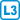## Number of parenthesizations

How many distinct arrangements are there of $$n$$ pairs of parentheses such that the parentheses can be correctly paired (i.e. good parenthesizations)?

• #### Hint

Try to describe the good (and bad) parenthesizations as paths in an $$n\times n$$ grid and then look for a property of the bad paths which will let you count them.

• #### Solution

We can represent a parenthesization of $$n$$ pairs of parentheses as a path of length $$2n$$ in an $$n\times n$$ grid (i.e. with $$n^2$$ squares) from the upper left corner to the lower right corner, where an opening parenthesis '(' indicates a step to the right and a closing parenthesis ')' indicates a step downward. The number of such paths, i.e. of such parenthesizations is $$\binom{2n}{n}$$

The bad parenthesizations are the ones which fall below the diagonal – at that moment the number of closing parenthses ')' used exceeds the number of opening parentheses '('.

We determine the number of bad paths as follows. We divide a bad path $$P$$ into two segments, namely segment $$A$$ from the start to the moment when the path falls below the diagonal, and the remaining segment $$B$$. We take the mirror image of segment $$A$$, yielding a segment $$A'$$, whose extension via $$B$$ yields a path $$P'$$.

This path $$P'$$ is in an $$(n-1)\times (n+1)$$ grid. The relationship $$P \leftrightarrow P'$$ is a bijection between bad paths in the original grid and all paths in the new grid, because we can also uniquely divide the path $$P'$$ into segments $$A'$$ a $$B$$. Segment $$A'$$ ends as soon as the path crosses to the right of a diagonal line leading from the upper left corner of the new grid. Such a moment must always occur, because the new grid is wider than it is long.

The number of possible parenthesizations is $$\binom{2n}{n}-\binom{2n}{n-1}=\frac{1}{n+1}\binom{2n}{n}$$.